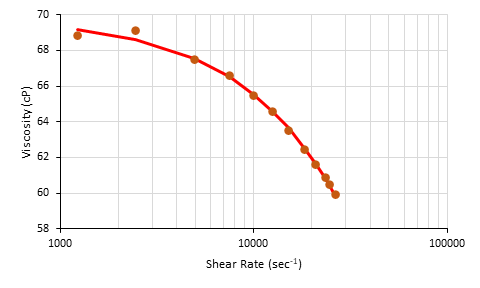# Application Note: Modeling non-Newtonian Fluids

Scientists have been studying non-Newtonian fluid behavior for decades and have developed models to facilitate characterization. The outputs of these models can be used to interpolate and extrapolate data, categorize materials based on model parameters, and help with computational fluid dynamics simulations. Two common models used to fit experimental non-Newtonian data are the Cross model and the Carreau-Yasuda model.

The Cross model is an empirical equation that is used to fit non-Newtonian data (Cross, 1979). The Cross model can describe many types of fluids including dispersions, polymer melts, and polymeric solutions.

The Carreau-Yasuda model is another empirical equation used to fit non-Newtonian data (Bird et al., 1987). It has more parameters than the Cross model and is the generalized form of the power law fluid model. The Carreau-Yasuda model is well suited for fluids that are beginning to shear thin, and can be used to describe emulsions, biopolymer solutions, protein solutions, polymer melts, and polymeric solutions.

In this application note, we will walk you through how to fit non-Newtonian data to both models using the Solver add-in of Microsoft Excel.